# 12.5 Conic sections in polar coordinates  (Page 6/8)

 Page 6 / 8

$\frac{{x}^{2}}{9}-\frac{{y}^{2}}{16}=1$

$\frac{{\left(y-1\right)}^{2}}{49}-\frac{{\left(x+1\right)}^{2}}{4}=1$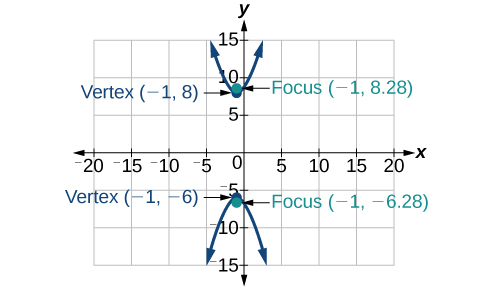${x}^{2}-4{y}^{2}+6x+32y-91=0$

$2{y}^{2}-{x}^{2}-12y-6=0$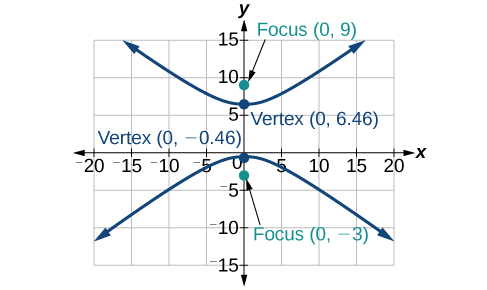For the following exercises, find the equation of the hyperbola.

Center at $\text{\hspace{0.17em}}\left(0,0\right),$ vertex at $\text{\hspace{0.17em}}\left(0,4\right),$ focus at $\text{\hspace{0.17em}}\left(0,-6\right)$

Foci at $\text{\hspace{0.17em}}\left(3,7\right)\text{\hspace{0.17em}}$ and $\text{\hspace{0.17em}}\left(7,7\right),$ vertex at $\text{\hspace{0.17em}}\left(6,7\right)$

$\frac{{\left(x-5\right)}^{2}}{1}-\frac{{\left(y-7\right)}^{2}}{3}=1$

## The Parabola

For the following exercises, write the equation of the parabola in standard form. Then give the vertex, focus, and directrix.

${y}^{2}=12x$

${\left(x+2\right)}^{2}=\frac{1}{2}\left(y-1\right)$

${\left(x+2\right)}^{2}=\frac{1}{2}\left(y-1\right);\text{\hspace{0.17em}}$ vertex: $\text{\hspace{0.17em}}\left(-2,1\right);\text{\hspace{0.17em}}$ focus: $\text{\hspace{0.17em}}\left(-2,\frac{9}{8}\right);\text{\hspace{0.17em}}$ directrix: $\text{\hspace{0.17em}}y=\frac{7}{8}$

${y}^{2}-6y-6x-3=0$

${x}^{2}+10x-y+23=0$

${\left(x+5\right)}^{2}=\left(y+2\right);\text{\hspace{0.17em}}$ vertex: $\text{\hspace{0.17em}}\left(-5,-2\right);\text{\hspace{0.17em}}$ focus: $\text{\hspace{0.17em}}\left(-5,-\frac{7}{4}\right);\text{\hspace{0.17em}}$ directrix: $\text{\hspace{0.17em}}y=-\frac{9}{4}$

For the following exercises, graph the parabola, labeling vertex, focus, and directrix.

${x}^{2}+4y=0$

${\left(y-1\right)}^{2}=\frac{1}{2}\left(x+3\right)$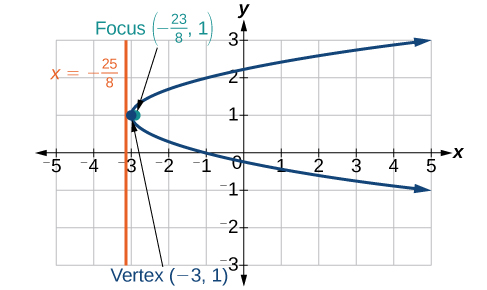${x}^{2}-8x-10y+46=0$

$2{y}^{2}+12y+6x+15=0$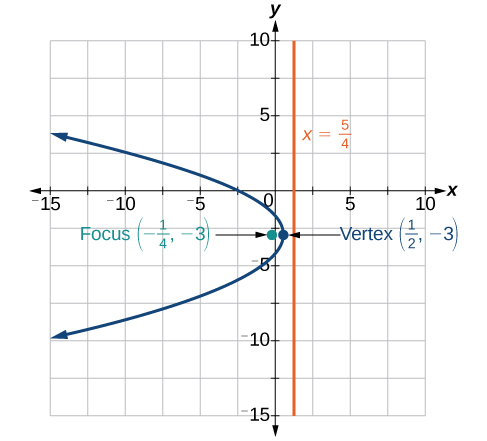For the following exercises, write the equation of the parabola using the given information.

Focus at $\text{\hspace{0.17em}}\left(-4,0\right);\text{\hspace{0.17em}}$ directrix is $\text{\hspace{0.17em}}x=4$

Focus at $\text{\hspace{0.17em}}\left(2,\frac{9}{8}\right);\text{\hspace{0.17em}}$ directrix is $\text{\hspace{0.17em}}y=\frac{7}{8}$

${\left(x-2\right)}^{2}=\left(\frac{1}{2}\right)\left(y-1\right)$

A cable TV receiving dish is the shape of a paraboloid of revolution. Find the location of the receiver, which is placed at the focus, if the dish is 5 feet across at its opening and 1.5 feet deep.

## Rotation of Axes

For the following exercises, determine which of the conic sections is represented.

$16{x}^{2}+24xy+9{y}^{2}+24x-60y-60=0$

${B}^{2}-4AC=0,$ parabola

$4{x}^{2}+14xy+5{y}^{2}+18x-6y+30=0$

$4{x}^{2}+xy+2{y}^{2}+8x-26y+9=0$

${B}^{2}-4AC=-31<0,$ ellipse

For the following exercises, determine the angle $\text{\hspace{0.17em}}\theta \text{\hspace{0.17em}}$ that will eliminate the $\text{\hspace{0.17em}}xy\text{\hspace{0.17em}}$ term, and write the corresponding equation without the $\text{\hspace{0.17em}}xy\text{\hspace{0.17em}}$ term.

${x}^{2}+4xy-2{y}^{2}-6=0$

${x}^{2}-xy+{y}^{2}-6=0$

$\theta ={45}^{\circ },{{x}^{\prime }}^{2}+3{{y}^{\prime }}^{2}-12=0$

For the following exercises, graph the equation relative to the $\text{\hspace{0.17em}}{x}^{\prime }{y}^{\prime }\text{\hspace{0.17em}}$ system in which the equation has no $\text{\hspace{0.17em}}{x}^{\prime }{y}^{\prime }\text{\hspace{0.17em}}$ term.

$9{x}^{2}-24xy+16{y}^{2}-80x-60y+100=0$

${x}^{2}-xy+{y}^{2}-2=0$

$\theta ={45}^{\circ }$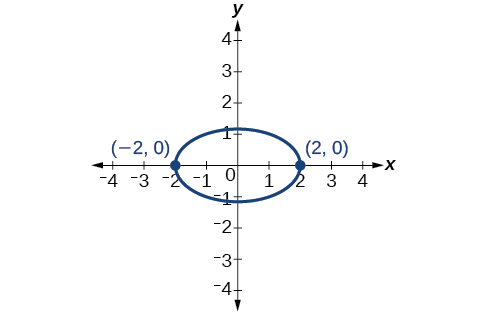$6{x}^{2}+24xy-{y}^{2}-12x+26y+11=0$

## Conic Sections in Polar Coordinates

For the following exercises, given the polar equation of the conic with focus at the origin, identify the eccentricity and directrix.

Hyperbola with $\text{\hspace{0.17em}}e=5\text{\hspace{0.17em}}$ and directrix $\text{\hspace{0.17em}}2\text{\hspace{0.17em}}$ units to the left of the pole.

Ellipse with $\text{\hspace{0.17em}}e=\frac{3}{4}\text{\hspace{0.17em}}$ and directrix $\text{\hspace{0.17em}}\frac{1}{3}\text{\hspace{0.17em}}$ unit above the pole.

For the following exercises, graph the conic given in polar form. If it is a parabola, label the vertex, focus, and directrix. If it is an ellipse or a hyperbola, label the vertices and foci.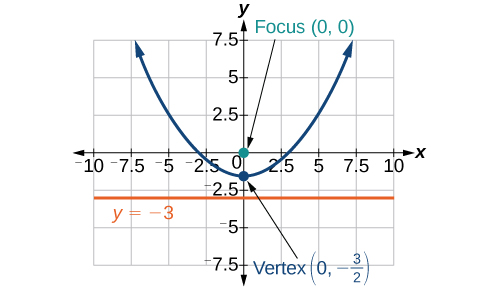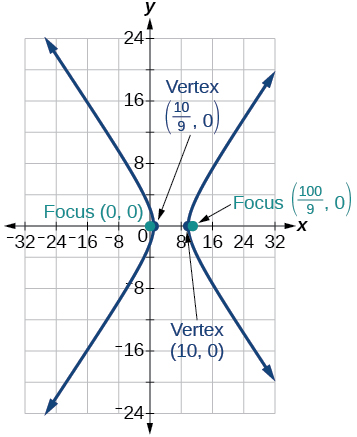For the following exercises, given information about the graph of a conic with focus at the origin, find the equation in polar form.

Directrix is $\text{\hspace{0.17em}}x=3\text{\hspace{0.17em}}$ and eccentricity $\text{\hspace{0.17em}}e=1$

Directrix is $\text{\hspace{0.17em}}y=-2\text{\hspace{0.17em}}$ and eccentricity $\text{\hspace{0.17em}}e=4$

## Practice test

For the following exercises, write the equation in standard form and state the center, vertices, and foci.

$\frac{{x}^{2}}{9}+\frac{{y}^{2}}{4}=1$

$\frac{{x}^{2}}{{3}^{2}}+\frac{{y}^{2}}{{2}^{2}}=1;\text{\hspace{0.17em}}$ center: $\text{\hspace{0.17em}}\left(0,0\right);\text{\hspace{0.17em}}$ vertices: $\text{\hspace{0.17em}}\left(3,0\right),\left(–3,0\right),\left(0,2\right),\left(0,-2\right);\text{\hspace{0.17em}}$ foci: $\left(\sqrt{5},0\right),\left(-\sqrt{5},0\right)$

$9{y}^{2}+16{x}^{2}-36y+32x-92=0$

For the following exercises, sketch the graph, identifying the center, vertices, and foci.

$\frac{{\left(x-3\right)}^{2}}{64}+\frac{{\left(y-2\right)}^{2}}{36}=1$

center: $\text{\hspace{0.17em}}\left(3,2\right);\text{\hspace{0.17em}}$ vertices: $\text{\hspace{0.17em}}\left(11,2\right),\left(-5,2\right),\left(3,8\right),\left(3,-4\right);\text{\hspace{0.17em}}$ foci: $\text{\hspace{0.17em}}\left(3+2\sqrt{7},2\right),\left(3-2\sqrt{7},2\right)$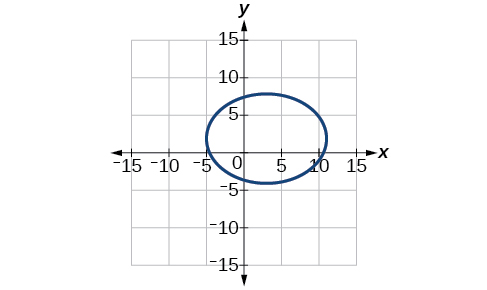$2{x}^{2}+{y}^{2}+8x-6y-7=0$

Write the standard form equation of an ellipse with a center at $\text{\hspace{0.17em}}\left(1,2\right),$ vertex at $\text{\hspace{0.17em}}\left(7,2\right),$ and focus at $\text{\hspace{0.17em}}\left(4,2\right).$

$\frac{{\left(x-1\right)}^{2}}{36}+\frac{{\left(y-2\right)}^{2}}{27}=1$

A whispering gallery is to be constructed with a length of 150 feet. If the foci are to be located 20 feet away from the wall, how high should the ceiling be?

For the following exercises, write the equation of the hyperbola in standard form, and give the center, vertices, foci, and asymptotes.

$\frac{{x}^{2}}{49}-\frac{{y}^{2}}{81}=1$

$\frac{{x}^{2}}{{7}^{2}}-\frac{{y}^{2}}{{9}^{2}}=1;\text{\hspace{0.17em}}$ center: $\text{\hspace{0.17em}}\left(0,0\right);\text{\hspace{0.17em}}$ vertices $\text{\hspace{0.17em}}\left(7,0\right),\left(-7,0\right);\text{\hspace{0.17em}}$ foci: $\text{\hspace{0.17em}}\left(\sqrt{130},0\right),\left(-\sqrt{130},0\right);\text{\hspace{0.17em}}$ asymptotes: $\text{\hspace{0.17em}}y=±\frac{9}{7}x$

$16{y}^{2}-9{x}^{2}+128y+112=0$

For the following exercises, graph the hyperbola, noting its center, vertices, and foci. State the equations of the asymptotes.

$\frac{{\left(x-3\right)}^{2}}{25}-\frac{{\left(y+3\right)}^{2}}{1}=1$

center: $\text{\hspace{0.17em}}\left(3,-3\right);\text{\hspace{0.17em}}$ vertices: $\text{\hspace{0.17em}}\left(8,-3\right),\left(-2,-3\right);$ foci: $\text{\hspace{0.17em}}\left(3+\sqrt{26},-3\right),\left(3-\sqrt{26},-3\right);\text{\hspace{0.17em}}$ asymptotes: $\text{\hspace{0.17em}}y=±\frac{1}{5}\left(x-3\right)-3$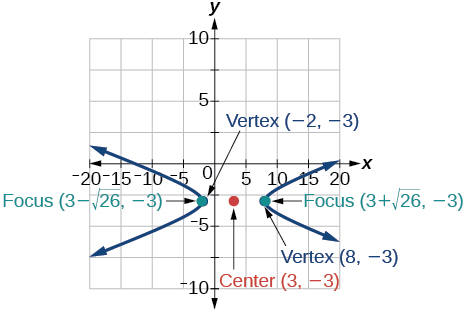${y}^{2}-{x}^{2}+4y-4x-18=0$

Write the standard form equation of a hyperbola with foci at $\text{\hspace{0.17em}}\left(1,0\right)\text{\hspace{0.17em}}$ and $\text{\hspace{0.17em}}\left(1,6\right),$ and a vertex at $\text{\hspace{0.17em}}\left(1,2\right).$

$\frac{{\left(y-3\right)}^{2}}{1}-\frac{{\left(x-1\right)}^{2}}{8}=1$

For the following exercises, write the equation of the parabola in standard form, and give the vertex, focus, and equation of the directrix.

${y}^{2}+10x=0$

$3{x}^{2}-12x-y+11=0$

${\left(x-2\right)}^{2}=\frac{1}{3}\left(y+1\right);\text{\hspace{0.17em}}$ vertex: $\text{\hspace{0.17em}}\left(2,-1\right);\text{\hspace{0.17em}}$ focus: $\text{\hspace{0.17em}}\left(2,-\frac{11}{12}\right);\text{\hspace{0.17em}}$ directrix: $\text{\hspace{0.17em}}y=-\frac{13}{12}$

For the following exercises, graph the parabola, labeling the vertex, focus, and directrix.

${\left(x-1\right)}^{2}=-4\left(y+3\right)$

${y}^{2}+8x-8y+40=0$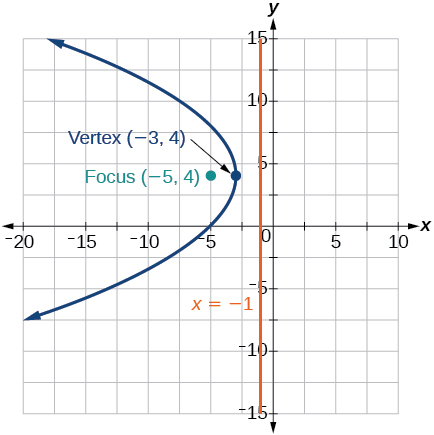Write the equation of a parabola with a focus at $\text{\hspace{0.17em}}\left(2,3\right)\text{\hspace{0.17em}}$ and directrix $\text{\hspace{0.17em}}y=-1.$

A searchlight is shaped like a paraboloid of revolution. If the light source is located 1.5 feet from the base along the axis of symmetry, and the depth of the searchlight is 3 feet, what should the width of the opening be?

Approximately $\text{\hspace{0.17em}}8.49\text{\hspace{0.17em}}$ feet

For the following exercises, determine which conic section is represented by the given equation, and then determine the angle $\text{\hspace{0.17em}}\theta \text{\hspace{0.17em}}$ that will eliminate the $\text{\hspace{0.17em}}xy\text{\hspace{0.17em}}$ term.

$3{x}^{2}-2xy+3{y}^{2}=4$

${x}^{2}+4xy+4{y}^{2}+6x-8y=0$

parabola; $\text{\hspace{0.17em}}\theta \approx {63.4}^{\circ }$

For the following exercises, rewrite in the $\text{\hspace{0.17em}}{x}^{\prime }{y}^{\prime }\text{\hspace{0.17em}}$ system without the $\text{\hspace{0.17em}}{x}^{\prime }{y}^{\prime }\text{\hspace{0.17em}}$ term, and graph the rotated graph.

$11{x}^{2}+10\sqrt{3}xy+{y}^{2}=4$

$16{x}^{2}+24xy+9{y}^{2}-125x=0$

${{x}^{\prime }}^{2}-4{x}^{\prime }+3{y}^{\prime }=0$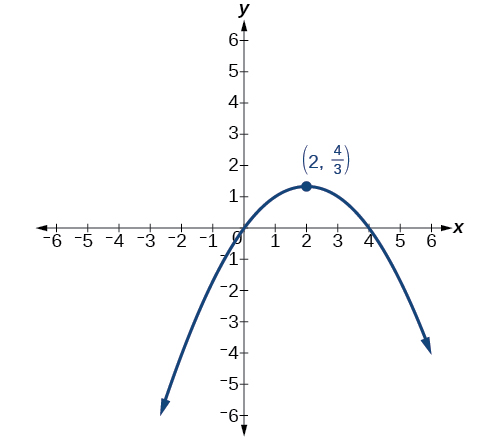For the following exercises, identify the conic with focus at the origin, and then give the directrix and eccentricity.

Hyperbola with $\text{\hspace{0.17em}}e=\frac{3}{2},\text{\hspace{0.17em}}$ and directrix $\text{\hspace{0.17em}}\frac{5}{6}\text{\hspace{0.17em}}$ units to the right of the pole.

For the following exercises, graph the given conic section. If it is a parabola, label vertex, focus, and directrix. If it is an ellipse or a hyperbola, label vertices and foci.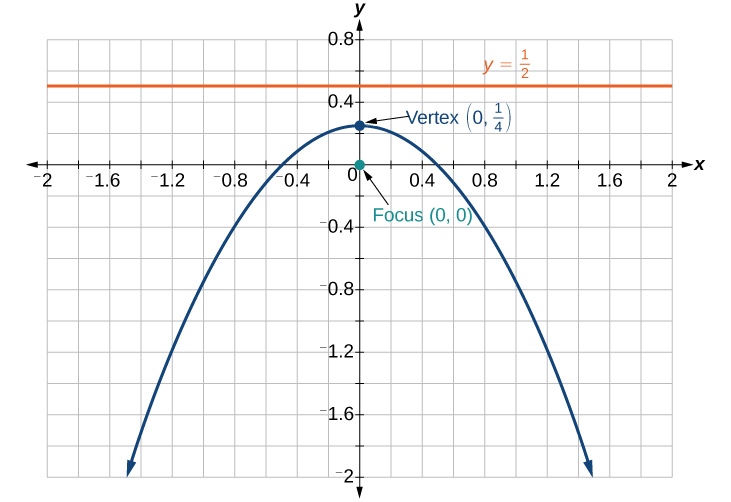Find a polar equation of the conic with focus at the origin, eccentricity of $\text{\hspace{0.17em}}e=2,$ and directrix: $\text{\hspace{0.17em}}x=3.$

(1+cosA+IsinA)(1+cosB+isinB)/(cos@+isin@)(cos$+isin$)
hatdog
Mark
how we can draw three triangles of distinctly different shapes. All the angles will be cutt off each triangle and placed side by side with vertices touching
bsc F. y algebra and trigonometry pepper 2
given that x= 3/5 find sin 3x
4
DB
remove any signs and collect terms of -2(8a-3b-c)
-16a+6b+2c
Will
Joeval
(x2-2x+8)-4(x2-3x+5)
sorry
Miranda
x²-2x+9-4x²+12x-20 -3x²+10x+11
Miranda
x²-2x+9-4x²+12x-20 -3x²+10x+11
Miranda
(X2-2X+8)-4(X2-3X+5)=0 ?
master
The anwser is imaginary number if you want to know The anwser of the expression you must arrange The expression and use quadratic formula To find the answer
master
The anwser is imaginary number if you want to know The anwser of the expression you must arrange The expression and use quadratic formula To find the answer
master
Y
master
master
Soo sorry (5±Root11* i)/3
master
Mukhtar
2x²-6x+1=0
Ife
explain and give four example of hyperbolic function
What is the correct rational algebraic expression of the given "a fraction whose denominator is 10 more than the numerator y?
y/y+10
Mr
Find nth derivative of eax sin (bx + c).
Find area common to the parabola y2 = 4ax and x2 = 4ay.
Anurag
y2=4ax= y=4ax/2. y=2ax
akash
A rectangular garden is 25ft wide. if its area is 1125ft, what is the length of the garden
to find the length I divide the area by the wide wich means 1125ft/25ft=45
Miranda
thanks
Jhovie
What do you call a relation where each element in the domain is related to only one value in the range by some rules?
A banana.
Yaona
given 4cot thither +3=0and 0°<thither <180° use a sketch to determine the value of the following a)cos thither
what are you up to?
nothing up todat yet
Miranda
hi
jai
hello
jai
Miranda Drice
jai
aap konsi country se ho
jai
which language is that
Miranda
I am living in india
jai
good
Miranda
what is the formula for calculating algebraic
I think the formula for calculating algebraic is the statement of the equality of two expression stimulate by a set of addition, multiplication, soustraction, division, raising to a power and extraction of Root. U believe by having those in the equation you will be in measure to calculate it
Miranda
state and prove Cayley hamilton therom
hello
Propessor
hi
Miranda
the Cayley hamilton Theorem state if A is a square matrix and if f(x) is its characterics polynomial then f(x)=0 in another ways evey square matrix is a root of its chatacteristics polynomial.
Miranda
hi
jai
hi Miranda
jai
thanks
Propessor
welcome
jai

#### Get Jobilize Job Search Mobile App in your pocket Now!ByBy Brooke DelaneyBy Saylor FoundationBy Jonathan LongBy OpenStaxBy Angela EckmanBy OpenStaxBy OpenStaxBy John GabrieliBy Jesenia WoffordBy Richley Crapo